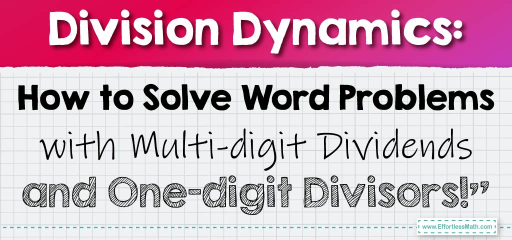# Division Dynamics: How to Solve Word Problems with Multi-digit Dividends and One-digit Divisors!”

When faced with real-world scenarios, we often need to divide multi-digit numbers by one-digit numbers. These word problems can range from sharing resources to distributing items among groups. Let's delve into some word problems that involve this type of division.## Dividing Multi-digit Numbers by One-digit Numbers in Word Problems

### Example 1:

Jenna has $$345$$ flyers to distribute for a community event. She wants to give them out in bundles of $$5$$. How many bundles will she have?

Solution Process:

Divide the total number of flyers by the number in each bundle: $$345 \div 5 = 69$$.

Jenna will have $$69$$ bundles.

The Absolute Best Book for 5th Grade Students

### Example 2:

A school cafeteria has $$256$$ sandwiches. They want to serve them equally in $$8$$ classrooms. How many sandwiches will each classroom get?

Solution Process:

Divide the total number of sandwiches by the number of classrooms: $$256 \div 8 = 32$$.

Each classroom will get $$32$$ sandwiches.

Dividing multi-digit numbers by one-digit numbers in word problems is a fundamental skill in mathematics. It’s essential for understanding how to distribute or group items equally. By carefully reading the problem, identifying the numbers involved, and performing the division, you can easily find the solution. Practice regularly with different scenarios to become proficient at tackling these real-world division challenges!

### Practice Questions:

1. A factory produces $$648$$ toys and wants to pack them in boxes of $$6$$. How many boxes will they need?

2. There are $$540$$ students in a school who need to be divided into groups of $$9$$ for a workshop. How many groups will there be?

3. A charity received $$738$$ donations and wants to organize them in piles of $$3$$. How many piles will they have?

4. A bookstore has $$462$$ books and wants to display them on $$6$$ shelves. How many books will each shelf have?

5. A farmer harvested $$980$$ potatoes and wants to sell them in bags of $$7$$. How many bags will he prepare?

A Perfect Book for Grade 5 Math Word Problems!

1. $$108$$ boxes

2. $$60$$ groups

3. $$246$$ piles

4. $$77$$ books per shelf

5. $$140$$ bags

The Best Math Books for Elementary Students

### What people say about "Division Dynamics: How to Solve Word Problems with Multi-digit Dividends and One-digit Divisors!” - Effortless Math: We Help Students Learn to LOVE Mathematics"?

No one replied yet.

X
51% OFF

Limited time only!

Save Over 51%

SAVE $15 It was$29.99 now it is \$14.99